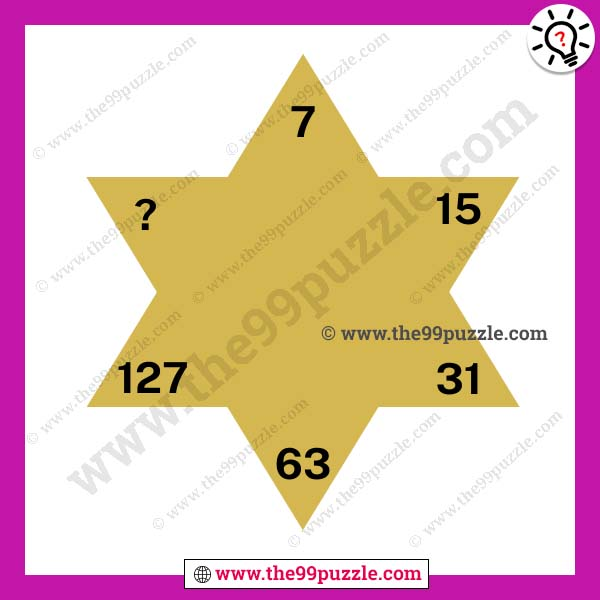# Brain twister star puzzles with answer – Puzz263

Math reasoning questions help many competitive aspirants who prepare for upcoming exams and interviews. In this brain twister star puzzles for genius where is your challenge to replace the question mark. All school students like these types of math puzzle questions. This fun and logical math puzzle make you smarter if you practice every day. In this mathematics, you have to find out the math tricks and replace the question mark. Genius students solve the puzzles very quickly.###### Explanation:

7+7 = 14+1 = 15

15+15=30+1=31

31+31=62+1=63

63+63=126+1=127

127+127=254+1=255